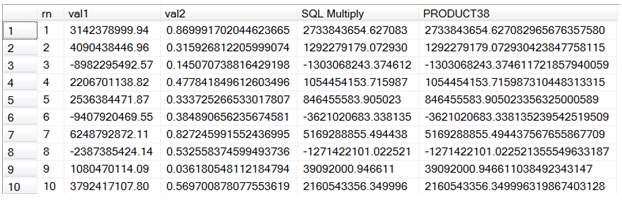# SQL Server PRODUCT function with precision 38 and scale 18

PRODUCT38

Updated: 30 November 2012

Use the scalar function PRODUCT38 to return the product of 2 decimal(38,18) values as a decimal with precision 38 and scale 18. Numeric is functionally equivalent to decimal.
Syntax
SELECT [wctMath].[wct].[PRODUCT38](
<@Val1, numeric(38,18),>
,<@Val2, numeric(38,18),>)
Arguments
@Val1
is the first value in the multiplication. @Val1 is an expression of type decimal(38,18) or of a type that can be implicitly converted to decimal(38,18)
@Val2
is the second value in the multiplication. @Val2 is an expression of type decimal(38,18) or of a type that can be implicitly converted to decimal(38,18)
Return Types
[numeric](38, 18)
Remarks
·         The maximum value returned by the function is 9999999999999999999.999999999999999999.
·         The minimum value returned by the function is -9999999999999999999.999999999999999999
·         If the product of @Val1 and @Val2 is greater than the maximum value or less than the minimum value, a NULL will be returned.
·         For division, use the QUOTIENT38 function.
Example
This example demonstrates the difference in results between standard decimal multiplication in SQL Server and the PRODUCT38 function.
CREATE TABLE #p(
rn       int,
val1     decimal(38,2),
val2     decimal(38,18)
)

INSERT INTO #p
SELECT *
FROM (VALUES
(1,3142378999.94000000000,0.869991702044623665),
(2,4090438446.96000000000,0.315926812205999074),
(3,-8982295492.57000000000,0.145070738816429198),
(4,2206701138.82000000000,0.477841849612603496),
(5,2536384471.87000000000,0.333725266533017807),
(6,-9407920469.55000000000,0.384890656235674581),
(7,6248792872.11000000000,0.827245991552436995),
(8,-2387385424.14000000000,0.532558374599493736),
(9,1080470114.09000000000,0.036180548112184794),
(10,3792417107.80000000000,0.569700878077553619)
) n(rn,val1, val2)

SELECT *
,val1 * val2 as [SQL Multiply]
,wct.PRODUCT38(Val1, val2) as PRODUCT38
FROM #p

DROP TABLE #p
This produces the following result.### SupportCopyright 2008-2021 Westclintech LLC         Privacy Policy        Terms of Service